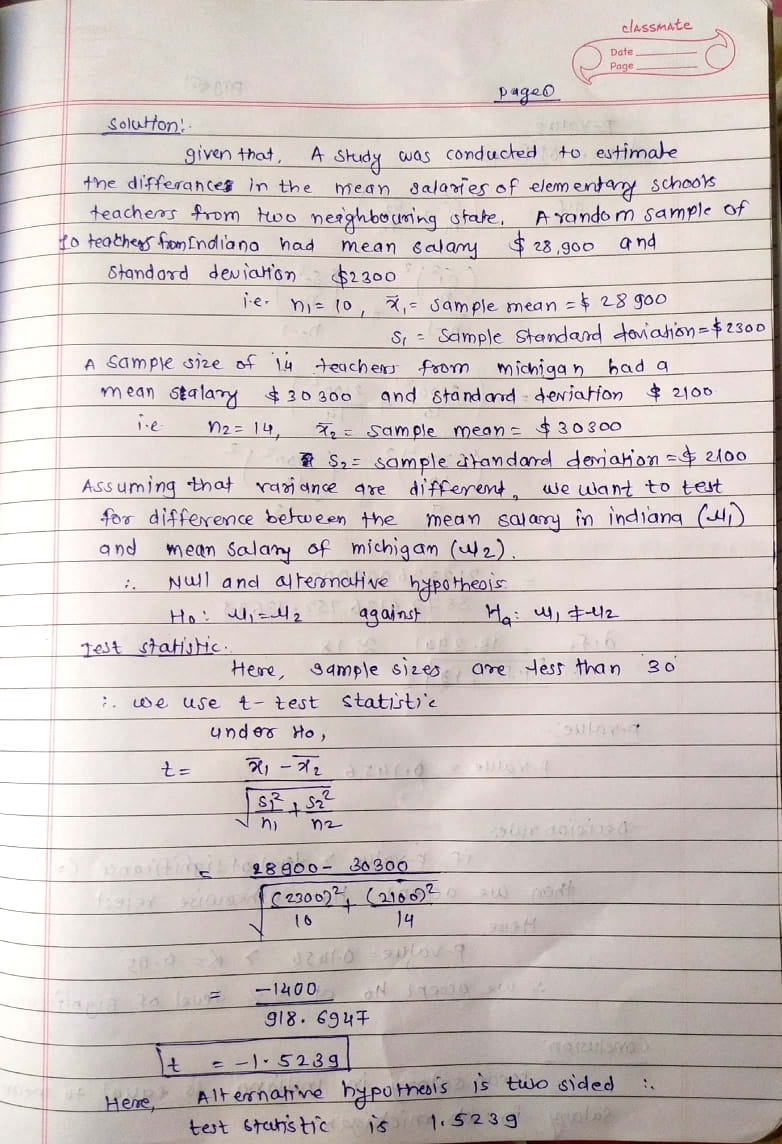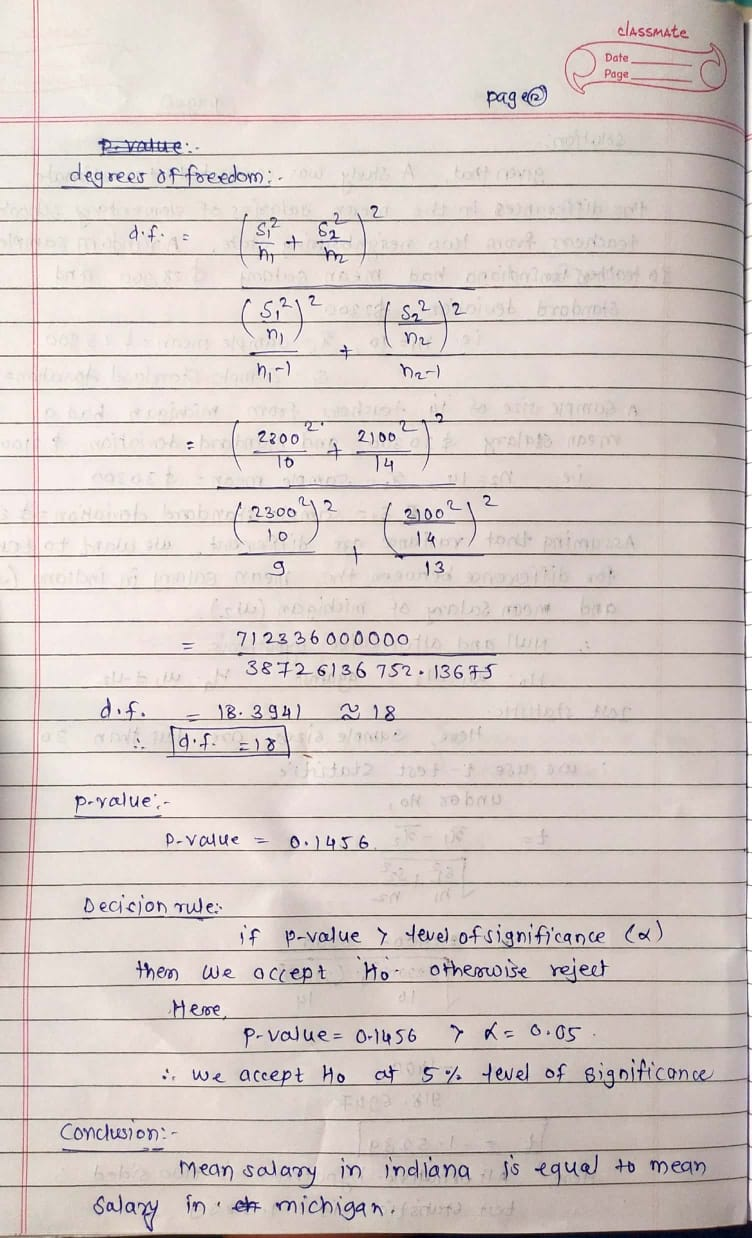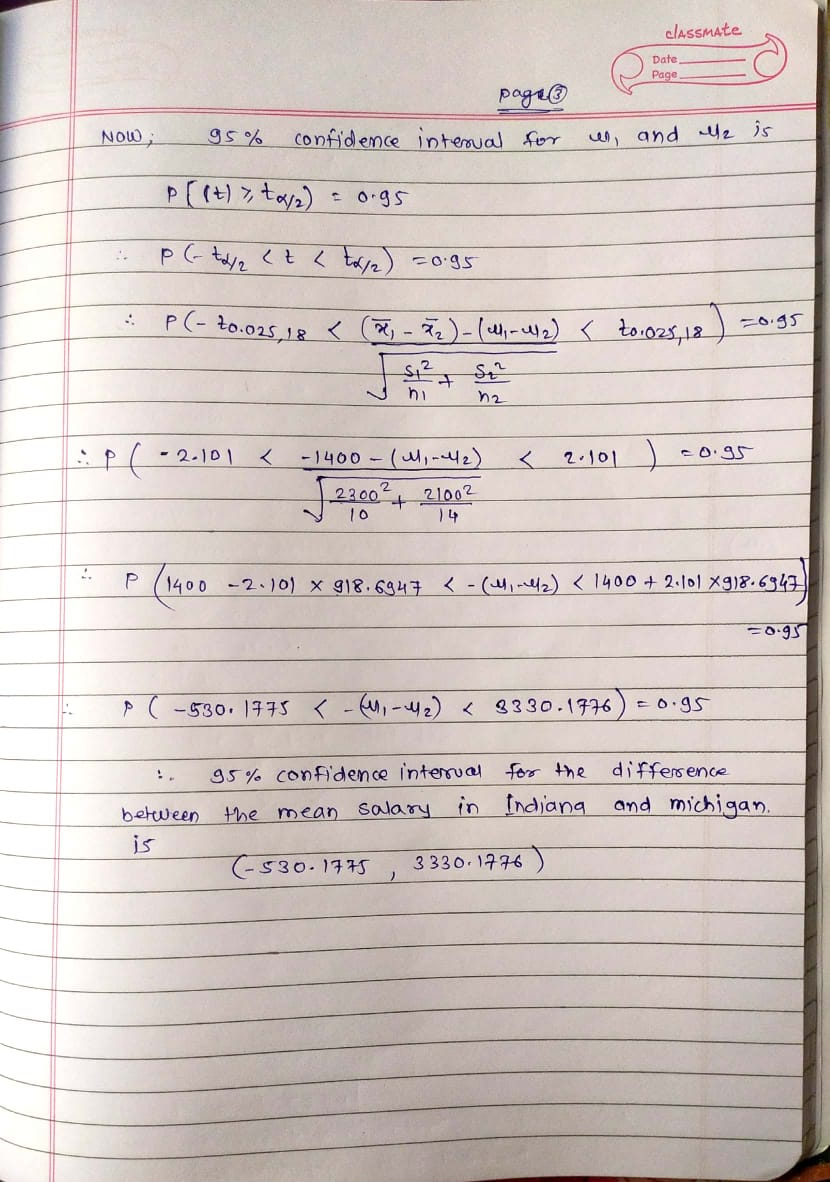##### Question

In: Statistics and Probability

# A study was conducted to estimate the difference in the mean salaries of elementary school teachers...

A study was conducted to estimate the difference in the mean salaries of elementary school teachers from two neighboring states. A sample of 10 teachers from the Indiana had a mean salary of $28,900 with a standard deviation of$2300. A sample of 14 teachers from Michigan had a mean salary of $30,300 with a standard deviation of$2100. Determine a 95% confidence interval for the difference between the mean salary in Indiana and Michigan.(Assume population variances are different.)

*Include null and alternate hypothesis, a p-value, and a conclusion PRIVATE about the null hypothesis*

## Solutions

##### Expert Solution

I hope this answer helps you....## Related Solutions

##### A study was conducted to determine if the salaries of elementary school teachers from two neighboring...
A study was conducted to determine if the salaries of elementary school teachers from two neighboring districts were equal. A sample of 15 teachers from each district was randomly selected. The mean from the first district was $28,900 with a standard deviation of$2300. The mean from the second district was $30,300 with a standard deviation of$2100. Assume the samples are random, independent, and come from populations that are normally distributed. Construct a 95% confidence interval for μ1 -...
##### Salaries for teachers in a particular elementary school district are normally distributed with a mean of...
Salaries for teachers in a particular elementary school district are normally distributed with a mean of $44,000 and a standard deviation of$6,500. We randomly survey ten teachers from that district. 1.Find the probability that the teachers earn a total of over $400,000 2.If we surveyed 70 teachers instead of ten, graphically, how would that change the distribution in part d? 3.If each of the 70 teachers received a$3,000 raise, graphically, how would that change the distribution in part...
##### A researcher claims that the mean of the salaries of elementary school teachers is greater than...
A researcher claims that the mean of the salaries of elementary school teachers is greater than the mean of the salaries of secondary school teachers in a large school district. The mean of the salaries of a random sample of 26 elementary school teachers is $48,250 and the sample standard deviation is$3900. The mean of the salaries of 24 randomly selected secondary school teachers is $45,630 with a sample standard deviation of$5530. At ? = 0.05, can it...
##### Salaries for teachers in a particular elementary school district are normally distributed with a mean of...
Salaries for teachers in a particular elementary school district are normally distributed with a mean of $44,000 and a standard deviation of$6,500. We randomly survey ten teachers from that district. Find the 85th percentile for the sum of the sampled teacher's salaries to 2 decimal places.